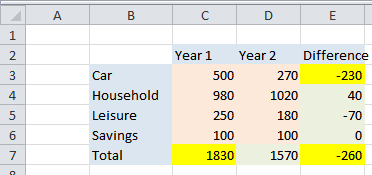## What is a spreadsheet?

Aug 132011

Many people have Microsoft Office but have never used the spreadsheet application (Excel) that is part of it. Every now and again someone asks me what a spreadsheet is, so I’d like to give an overview. This is not a tutorial for financial or computer geeks: it’s just to give you an idea of whether you think spreadsheets could be worth investigating further.

A spreadsheet is a computer application that stores and calculates text and figures on documents that are like sheets of paper divided into rows and columns. These “sheets of paper” can then be saved in much the same way as Word documents.Excel Spreadsheet - figure 1

Each row and each column of the spreadsheet is labelled (with letters for columns and numbers for rows). Therefore, each individual box formed by the conjunction of a row and a column (called a cell) will have its own unique address – eg C2 or F19. Rows go down the page and columns go across the page.

Looking at Figure 1:-

• The cell that is labelled C2 contains a piece of text (“Year 1”). It is actually possible to perform calculations on pieces of text but in most cases – as here – the text in the cell C2 simply labels the data that appears below it.
• The cell C3 has a number in it (200), as do the rows below it.
• The cell C7 contains a calculation. In this case, the calculation tells the spreadsheet to add up the contents of the cells in the rows above and to place the answer in the cell C7. The actual calculation placed in the cell in this case is “=sum(C3:C6)”.
• The calculation in cell E3 tells the spreadsheet to subtract the contents of C3 from the contents of D3. The actual calculation is “C3-D3”.

For the sake of clarity I have colour-coded the cells in this example. Blue cells are text, orange cells are numbers, green cells are calculations. We enter text, numbers and dates just by typing in the data. To enter a calculation we begin by typing the “=” sign and then enter the formula.

Now, the beauty of spreadsheets is that having created this structure we can change any of the data and all of the formulae will be re-calculated immediately. So, for example, if we change the 200 in C3 to 500, then the totals immediately change as highlighted in yellow in figure 2 below:Excel Spreadsheet - figure 2

This means that we can create a structure that we want to use time and time again but only have to create that structure once. So, I might create the following structure (figure 3) and save it with the name of “expenditure template”:Excel Spreadsheet - figure 3

This template has the text and calculations in place but no actual figures. When I want to put in figures I open this spreadsheet, enter my figures, and then save it with a different name (using the “save as” command) so that I still have my empty template available to repeat the process in the future and also have a saved copy of the spreadsheets that include my figures. I can, of course, do this as often as necessary (eg monthly).

Spreadsheets can range from the very simple to the enormously complicated. The calculations I showed above include just the instruction (known as a function) to “SUM” (ie “add”) the contents of some specified cells, and the simple arithmetic operation of substracting the contents of one cell from another. There are many in-built functions and operators that can handle, for instance, date arithmetic, statistical functions, logical comparisons etc, but you don’t need to be intimidated by all this power. It is fairly simple to grasp enough of the concepts and techniques to handle most daily requirements.

Something it’s difficult to appreciate in this static article is that it is easier to create the structure and the calculations than you might think at first. This is mainly for three reasons:

• We can select the cells we wish to include in the calculation by “pointing” at them rather than manually typing in the cell co-ordinates.
• Once we have created an initial calculation we can “copy” that calculation to other rows or columns where that makes sense. For instance, having created the calculation in E3 (where the calculation is “D3-C3”) we can just copy that calculation down to the next four rows. The program will automatically adjust the cell references (eg “D4-C4”, “D5-C5” etc) as it makes the copies.
• Rows and cells can be inserted and deleted and the contents can be moved around as well as copied. The spreadsheet will automatically make changes in the calculations to adjust for these changes. This means that the design process can be very fluid: we don’t have to get it right first time.

A slightly different use of spreadsheets is to keep a kind of “database” of information (although I hesitate to use the word database as that has a more specifc meaning in computer terms). For instance, you could have a list of names, addresses, telephone numbers, email addresses etc in which each record (each person) is contained on one row and each different piece of information is in a different column (eg name, landline number, mobile number). This kind of list also has the advantage that in a modern spreadsheet application such as Excel, email addresses and website addresses are automatically recognised as links so you can click on them to create emails or go directly to websites (actually, the email part of that statement won’t work if you only have webmail on your computer).

Some of the different spreadsheets that I have cover the following uses:

• Comparing budget (or target) figures with actual figures.
• Comparing expenditure between different time periods.
• Keeping simple lists of items with values and their totals.